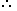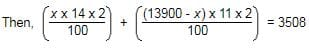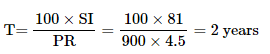UPSC  >  Test: Simple Interest- 1

# Test: Simple Interest- 1 - UPSC

Test Description

## 10 Questions MCQ Test CSAT Preparation - Test: Simple Interest- 1

Test: Simple Interest- 1 for UPSC 2023 is part of CSAT Preparation preparation. The Test: Simple Interest- 1 questions and answers have been prepared according to the UPSC exam syllabus.The Test: Simple Interest- 1 MCQs are made for UPSC 2023 Exam. Find important definitions, questions, notes, meanings, examples, exercises, MCQs and online tests for Test: Simple Interest- 1 below.
Solutions of Test: Simple Interest- 1 questions in English are available as part of our CSAT Preparation for UPSC & Test: Simple Interest- 1 solutions in Hindi for CSAT Preparation course. Download more important topics, notes, lectures and mock test series for UPSC Exam by signing up for free. Attempt Test: Simple Interest- 1 | 10 questions in 10 minutes | Mock test for UPSC preparation | Free important questions MCQ to study CSAT Preparation for UPSC Exam | Download free PDF with solutions
 1 Crore+ students have signed up on EduRev. Have you?
Test: Simple Interest- 1 - Question 1

### Suresh for 2 years invested Rs. 500 in SBI. He also invested Rs. 300 in ICICI for 4 years. At the end he received Rs. 220 from both banks as simple interest. What must have been rate of interest? (assuming the rate of interest for both banks is same)

Detailed Solution for Test: Simple Interest- 1 - Question 1

Simple Intrest = SI = PRT/100
Where P = Principal, R = Rate of intrest and T = time period
Total SI = SI from SBI and SI from ICICI
∴ 220 = (500 x R x 2)/100 + (300 x R x 4)/100
⇒ 220 = 10R + 12R
⇒ R = 10% = Rate of intrest

Test: Simple Interest- 1 - Question 2

### A sum of money at simple interest amounts to Rs. 815 in 3 years and to Rs. 854 in 4 years. The sum is :

Detailed Solution for Test: Simple Interest- 1 - Question 2

S.I. for 1 year = Rs. (854 - 815) = Rs. 39.

S.I. for 3 years = Rs.(39 * 3) = Rs. 117.Principal = Rs. (815 - 117) = Rs. 698.

Test: Simple Interest- 1 - Question 3

### A man took loan from a bank at the rate of 8% p.a. simple interest. After 4 years he had to pay Rs. 6200 interest only for the period. The principal amount borrowed by him was:

Detailed Solution for Test: Simple Interest- 1 - Question 3

SI = PRT / 100
⇒ (SI x 100) / RT = P
⇒ P = (6200*100) / 32
⇒ P = 19375

Test: Simple Interest- 1 - Question 4

Mr. Thomas invested an amount of Rs. 13,900 divided in two different schemes A and B at the simple interest rate of 14% p.a. and 11% p.a. respectively. If the total amount of simple interest earned in 2 years be Rs. 3508, what was the amount invested in Scheme B?

Detailed Solution for Test: Simple Interest- 1 - Question 4

Let the sum invested in Scheme A be Rs. x and that in Scheme B be Rs. (13900 - x)⇒ 28x - 22x = 350800 - (13900 * 22)
⇒ 6x = 45000
⇒ x = 7500
So, sum invested in Scheme B = Rs. (13900 - 7500) = Rs. 6400

Test: Simple Interest- 1 - Question 5

A sum fetched a total simple interest of Rs. 929.20 at the rate of 8 p.c.p.a. in 5 years. What is the sum?

Detailed Solution for Test: Simple Interest- 1 - Question 5

Given, SI = Rs 929.20
P = ?
T = 5 years
R = 8%
P = (100 x SI) / RT
⇒ (100 x 929.20) / (8 x 5)
⇒ Rs. 2323

Test: Simple Interest- 1 - Question 6

Arun took a loan of Rs. 1400 with simple interest for as many years as the rate of interest. If he paid Rs.686 as interest at the end of the loan period, what was the rate of interest?

Detailed Solution for Test: Simple Interest- 1 - Question 6

Simple Interest (SI) = P N R / 100

P is the Principal loan amount = Rs.1400

N is the number of years of deposit

R is the rate of interest

It is given that the loan period is as many years as the rate of interest.

So, N = R

Interest at the end of the loan period (SI ) = Rs.686

So,

686 = 1400 * R * R /100

R2 = 686*100 /1400

R2 = 49

R = 7%

Test: Simple Interest- 1 - Question 7

What will be the ratio of simple interest earned by certain amount at the same rate of interest for 5 years and that for 15 years?

Detailed Solution for Test: Simple Interest- 1 - Question 7

Simple Interest = PRT / 100
Here P = Principal, R = Rate of interest and T = time period
Hence, Simple Interest ∝ T
Required Ratio = (Simple Interest for 5 years) / (Simple Interest for 15 years)
⇒ T1/ T2 = 5 / 15
⇒ 1 / 3 = 1 : 3

Test: Simple Interest- 1 - Question 8

A sum of money amounts to Rs.9800 after 5 years and Rs.12005 after 8 years at the same rate of simple interest. The rate of interest per annum is

Detailed Solution for Test: Simple Interest- 1 - Question 8

We can get SI of 3 years = 12005 - 9800 = 2205

SI for 5 years = (2205/3)*5 = 3675 [so that we can get principal amount after deducting SI]

Principal = 9800 - 3675 = 6125

So Rate = (100*3675)/(6125*5) = 12%

Test: Simple Interest- 1 - Question 9

Q. How much time will it take for an amount of Rs. 900 to yield Rs. 81 as interest at 4.5% per annum of simple interest?

Detailed Solution for Test: Simple Interest- 1 - Question 9

Given, P = Rs.900

SI = Rs.81

T = ?

R = 4.5%Test: Simple Interest- 1 - Question 10

A certain amount earns simple interest of Rs. 1750 after 7 years. Had the interest been 2% more, how much more interest would it have earned?

Detailed Solution for Test: Simple Interest- 1 - Question 10

We need to know the S.I, principal and time to find the rate. Since the principal is not given, so data is inadequate.

## CSAT Preparation

197 videos|151 docs|200 tests
Information about Test: Simple Interest- 1 Page
In this test you can find the Exam questions for Test: Simple Interest- 1 solved & explained in the simplest way possible. Besides giving Questions and answers for Test: Simple Interest- 1, EduRev gives you an ample number of Online tests for practice

## CSAT Preparation

197 videos|151 docs|200 tests

### How to Prepare for UPSC

Read our guide to prepare for UPSC which is created by Toppers & the best Teachers(Scan QR code)